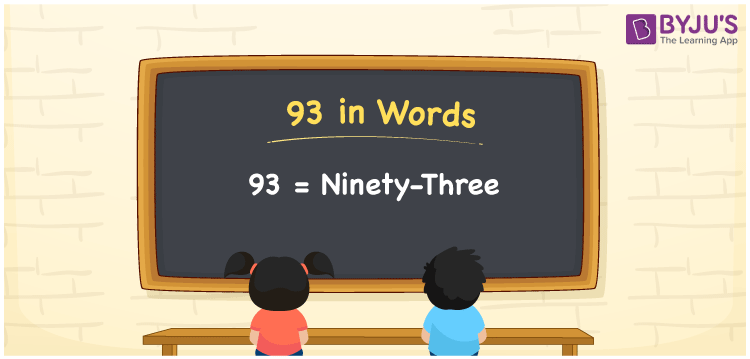# 93 in Words

93 in words can be written as Ninety-three. If you buy a dish at the restaurant for Rs. 93, then you can say that “I bought a dish for Ninety-three Rupees”. In this article, students will get a clear cut knowledge of the importance of numbers and how to count it. To write numbers in words, the faculty have made use of the English alphabet. Therefore, the number 93 can be read as “Ninety-three” in words.

 93 in words Ninety-three Ninety-three in Numbers 93

## 93 in English Words## How to Write 93 in Words?

The concept of a place value chart for 93 in words is explained in simple language. Two digits are present in the number 93. The place value chart indicating the place value of two digits is mentioned here.

 Tens Ones 9 3

The expanded form of 93 is provided below:

9 × Ten + 3 × One

= 9 × 10 + 3 × 1

= 90 + 3

= 93

= Ninety-three

Therefore, 93 in words is written as Ninety-three.

93 is a natural number that precedes 94 and succeeds 92.

93 in words – Ninety-three

Is 93 an odd number? – Yes

Is 93 an even number? – No

Is 93 a perfect square number? – No

Is 93 a perfect cube number? – No

Is 93 a prime number? – No

Is 93 a composite number? – Yes

## Frequently Asked Questions on 93 in Words

### How do you write the number 93 in words?

93 in words is Ninety-three.

### Find the value of 100 minus 7.

The value of 100 minus 7 is 93. Therefore, 93 in words is Ninety-three.

### Is 93 a perfect square?

No, 93 is not a perfect square as it cannot be expressed as the product of two integers which are equal.